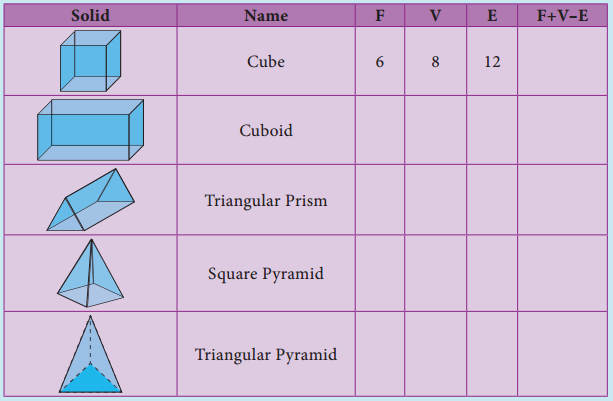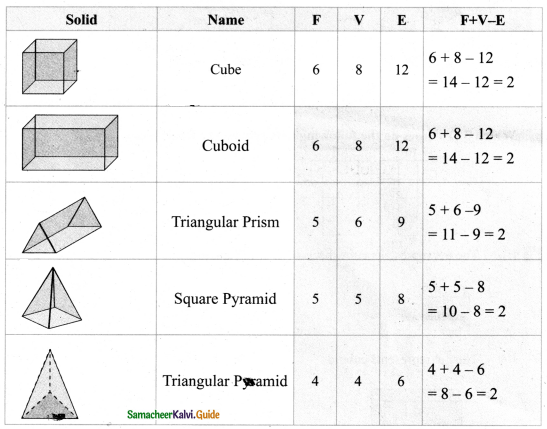Tamilnadu State Board New Syllabus Samacheer Kalvi 8th Maths Guide Pdf Chapter 2 Measurements InText Questions Text Book Back Questions and Answers, Notes.

## Tamilnadu Samacheer Kalvi 8th Maths Solutions Chapter 2 Measurements InText Questions

Think (Text Book Page No. 51)

Question 1.
$$\frac{22}{7}$$ and 3.14 are rational numbers. Is ‘π’ a rational number? Why?
$$\frac{22}{7}$$ and 3.14 are rational numbers π has non-terminating and non-repoating decimal expansion. So it is not a rational number. it is an irrational number.Question 2.
When is the’π’day celebrated? Why?
March 14th i.e. 3/14 every year. Approximately value of’ ‘π’ is 3.14.

Think (Text Book Page No. 53)

The given circular figure is di4dd into six equal parts. Can we call the parts as sectors? Why?No, the equal parts are not sectors. Because a sector is a plane surface that is enclosed between two radii and the circular arc of the circle. Here the boundaries are not radii.Try These (Text Book Page No. 53)

Fill the central angle of the shaded sector (each circle is divided into equal sectors)Think (Text Book Page No. 54)

Question 1.
Instead of multiplying by $$\frac{1}{2}$$, $$\frac{1}{3}$$ and $$\frac{1}{4}$$, we shall multiply by $$\frac{180^{\circ}}{360^{\circ}}$$, $$\frac{120^{\circ}}{360^{\circ}}$$ and $$\frac{90^{\circ}}{360^{\circ}}$$ respectively. why?
So, $$\frac{180^{\circ}}{360^{\circ}}$$ = $$\frac{1}{2}$$
$$\frac{120^{\circ}}{360^{\circ}}$$ = $$\frac{1}{3}$$
$$\frac{90^{\circ}}{360^{\circ}}$$ = $$\frac{1}{4}$$

Think (Text Book Page No. 57)

If the radius of a circle is doubled, what will happen to the area of the new circle so formed?
If r = 2r1 ⇒ Area of the circle = πr2 = π(2r1)2 = π4r12
Area = 4 × old area

Think (Text Book Page No. 61)

All the sides of a rhombus are equal. It is a regular polygon?
For a regular polygon all sides and all the angles must be equal. But in a rhombus all the sides are equal. But all the angles are not equal
∴ It is not a regular polygon.Try This (Text Book Page No. 64)

In the above example split the given mat into two trapeziums and verify your answer.
Area of the mat = Area of I trapezium + Area of II trapezium
= [$$\frac { 1 }{ 2 }$$ × h1 × (a1 + b1)] + [$$\frac { 1 }{ 2 }$$ × h2 × (a2 + b2)] sq. units
= [$$\frac { 1 }{ 2 }$$ × 2 × (7 + 5)] + $$\frac { 1 }{ 2 }$$ × 2 × (9 + 7) sq.feet
= 12 + 16 = 28 sq.feet
∴ Cost per sq.feet = ₹ 20
Cost for 28sq. feet = ₹ 20 × 28 = ₹ 560
∴ Total cost for the entire mat = ₹ 560
Both the answers are the same.Try This (Text Book Page No. 68)

Tabulate the number of faces (F), vertices (V) and edges (E) for the following polyhedrons. Also find F + V – EWhat do you observe from the above table? We observe that, F + V – E = 2 in all the cases. This is true for any polyhedron and this relation F + V – E = 2 is known as Euler’s formula.